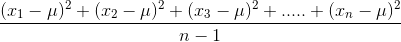# Problem 45779. Variance computation (★★★)

Given a vector x with several values, compute the variance, whose formula is given by:where x1, x2, ...., xn are the n elements of the vector x, and μ is the average of the vector x.
For example, if
x = [-1, 3, 5, -4, 10, 12]
Then μ=4.16667 the variance is 38.16667. The following commands are forbidden: std, var, mean, for, while.

### Solution Stats

28.69% Correct | 71.31% Incorrect
Last Solution submitted on Oct 27, 2022

### Community Treasure Hunt

Find the treasures in MATLAB Central and discover how the community can help you!

Start Hunting!